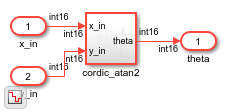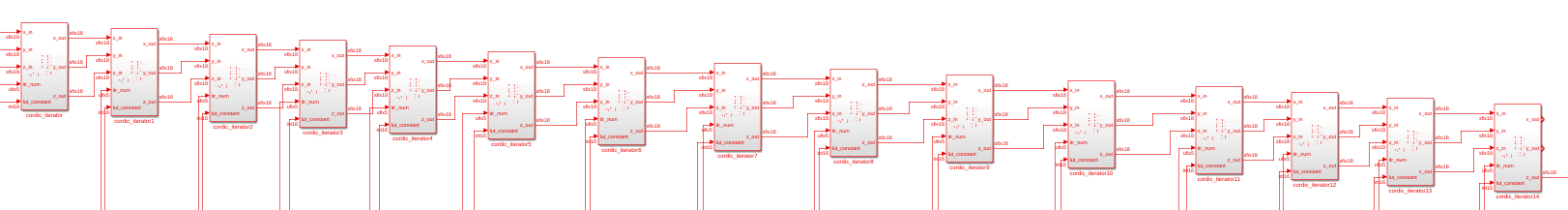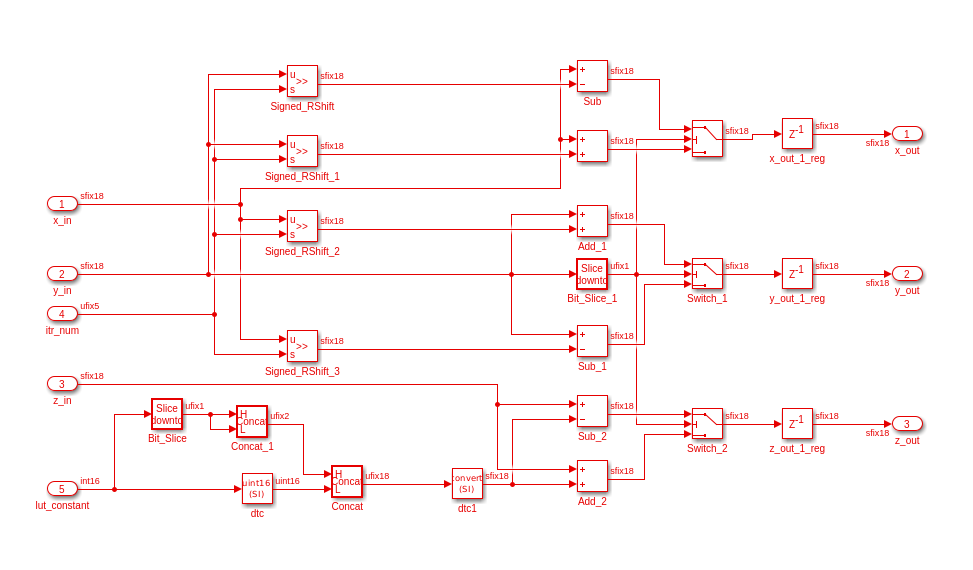# Generate Simulink Model from CORDIC Atan2 Verilog Code

This example shows how you can import a file containing Verilog® code and generate the corresponding Simulink® model by using the `importhdl` function. `importhdl` imports and parses the specified Verilog files to generate the corresponding Simulink model. The Verilog code in this example contains a CORDIC atan2 algorithm.

### CORDIC 2-Argument Arctangent (atan2) Verilog Design

```cordic_atan2_verilog_file = 'cordic_atan2.v'; type(cordic_atan2_verilog_file); ```
````timescale 1ns / 1ps module cordic_atan2( input clk, // clock input reset, // reset to the system input enable, // enable input signed [15:0] x_in, // Input x value input signed [15:0] y_in, // Input Y value output reg signed [15:0] theta // Input theta value ); // Pipeline the input values reg signed [17:0]x_in_d; reg signed [17:0]y_in_d; always @(posedge clk) begin if(reset) begin x_in_d <={18{1'b0}}; y_in_d <={18{1'b0}}; end else if(enable) begin // extend the input values for intermediate calculations x_in_d <= {{2{x_in}},x_in}; y_in_d <= {{2{y_in}},y_in}; end end // pre quad correction logic reg x_qaud_adjust; reg y_qaud_adjust; reg y_non_zero; reg signed [17:0]x_pre_quad_out; reg signed [17:0]y_pre_quad_out; always @(posedge clk)begin if(reset)begin x_pre_quad_out <= {18{1'b0}}; y_pre_quad_out <= {18{1'b0}}; x_qaud_adjust <= 1'b0; y_qaud_adjust <= 1'b0; y_non_zero <=1'b0; end else if(enable)begin if(x_in_d == 1'b1)begin x_pre_quad_out <= -x_in_d; x_qaud_adjust <= 1'b1; end else begin x_pre_quad_out <= x_in_d; x_qaud_adjust <= 1'b0; end if(y_in_d == 1'b1)begin y_pre_quad_out <= -y_in_d; y_qaud_adjust <= 1'b1; y_non_zero <=1'b1; end else begin y_pre_quad_out <= y_in_d; y_qaud_adjust <= 1'b0; y_non_zero <= |y_in_d; // reduction or for test non zero or not end end end //LOOKUP TABLE FOR THE ANGLES wire signed [15:0]LUT[0:14]; wire signed [17:0]x_k[0:15]; wire signed [17:0]y_k[0:15]; wire signed [17:0]z_k[0:15]; wire signed [15:0]theta_temp; parameter PI = 16'sh6488; assign LUT = 16'h1922; assign LUT = 16'h0Ed6; assign LUT = 16'h07D7; assign LUT = 16'h03FB; assign LUT = 16'h01FF; assign LUT = 16'h0100; assign LUT = 16'h0080; assign LUT = 16'h0040; assign LUT = 16'h0020; assign LUT = 16'h0010; assign LUT = 16'h0008; assign LUT = 16'h0004; assign LUT = 16'h0002; assign LUT = 16'h0001; assign LUT = 16'h0000; assign x_k = x_pre_quad_out; assign y_k = y_pre_quad_out; wire signed [17:0]theta_temp0; assign theta_temp0 = z_k; assign theta_temp = theta_temp0[15:0]; assign z_k = 18'd0; //cordic iterator genvar i; generate for (i = 0; i<15; i =i+1) begin Kernel cordic_iterator (.clk (clk), .reset(reset), .enable (enable ), .itr_num(i), .x_in(x_k[i]), .y_in(y_k[i]), .z_in(z_k[i]), .lut_constant(LUT[i]), .x_out(x_k[i+1]), .y_out(y_k[i+1]), .z_out(z_k[i+1]) ); end endgenerate // matching delays for the control signls of the pre quadrant correction logic reg x_qaud_adjust_match_delay[0:14]; reg y_qaud_adjust_match_delay[0:14]; reg y_non_zero_match_delay[0:14]; integer k; always @(posedge clk)begin if(reset)begin x_qaud_adjust_match_delay <= 1'b0; y_qaud_adjust_match_delay <= 1'b0; y_non_zero_match_delay  <= 1'b0; x_qaud_adjust_match_delay <= 1'b0; y_qaud_adjust_match_delay <= 1'b0; y_non_zero_match_delay  <= 1'b0; x_qaud_adjust_match_delay <= 1'b0; y_qaud_adjust_match_delay <= 1'b0; y_non_zero_match_delay  <= 1'b0; x_qaud_adjust_match_delay <= 1'b0; y_qaud_adjust_match_delay <= 1'b0; y_non_zero_match_delay  <= 1'b0; x_qaud_adjust_match_delay <= 1'b0; y_qaud_adjust_match_delay <= 1'b0; y_non_zero_match_delay  <= 1'b0; x_qaud_adjust_match_delay <= 1'b0; y_qaud_adjust_match_delay <= 1'b0; y_non_zero_match_delay  <= 1'b0; x_qaud_adjust_match_delay <= 1'b0; y_qaud_adjust_match_delay <= 1'b0; y_non_zero_match_delay  <= 1'b0; x_qaud_adjust_match_delay <= 1'b0; y_qaud_adjust_match_delay <= 1'b0; y_non_zero_match_delay  <= 1'b0; x_qaud_adjust_match_delay <= 1'b0; y_qaud_adjust_match_delay <= 1'b0; y_non_zero_match_delay  <= 1'b0; x_qaud_adjust_match_delay <= 1'b0; y_qaud_adjust_match_delay <= 1'b0; y_non_zero_match_delay  <= 1'b0; x_qaud_adjust_match_delay <= 1'b0; y_qaud_adjust_match_delay <= 1'b0; y_non_zero_match_delay  <= 1'b0; x_qaud_adjust_match_delay <= 1'b0; y_qaud_adjust_match_delay <= 1'b0; y_non_zero_match_delay  <= 1'b0; x_qaud_adjust_match_delay <= 1'b0; y_qaud_adjust_match_delay <= 1'b0; y_non_zero_match_delay  <= 1'b0; x_qaud_adjust_match_delay <= 1'b0; y_qaud_adjust_match_delay <= 1'b0; y_non_zero_match_delay  <= 1'b0; x_qaud_adjust_match_delay <= 1'b0; y_qaud_adjust_match_delay <= 1'b0; y_non_zero_match_delay  <= 1'b0; end else if(enable) begin x_qaud_adjust_match_delay <=x_qaud_adjust; y_qaud_adjust_match_delay <=y_qaud_adjust; y_non_zero_match_delay <=y_non_zero; for(k =0; k <14 ;k = k+1)begin x_qaud_adjust_match_delay[k+1] <= x_qaud_adjust_match_delay[k]; y_qaud_adjust_match_delay[k+1] <= y_qaud_adjust_match_delay[k]; y_non_zero_match_delay[k+1] <= y_non_zero_match_delay[k]; end end end // post quadrant correction logic always @(posedge clk) begin if(reset) theta<=16'd0; else if(enable)begin if(y_non_zero_match_delay)begin if(x_qaud_adjust_match_delay)begin if(y_qaud_adjust_match_delay) theta <=theta_temp -PI; else theta <=PI -theta_temp; end else begin if(y_qaud_adjust_match_delay) theta <= -theta_temp; else theta <= theta_temp; end end else if(x_qaud_adjust_match_delay)begin theta <= PI; end else begin theta <= 16'd0; end end end endmodule module Kernel( input clk, input reset, input enable, input signed [17:0]x_in, input signed [17:0]y_in, input signed [17:0]z_in, input [4:0]itr_num, input signed [15:0]lut_constant, output reg signed [17:0]x_out, output reg signed [17:0]y_out, output reg signed [17:0]z_out); wire signed [17:0] lut_constant_signExtension = {{2{lut_constant}},lut_constant}; always @(posedge clk)begin if(reset)begin x_out <={18{1'b0}}; y_out <={18{1'b0}}; z_out <= {18{1'b0}}; end else if(enable)begin if(y_in)begin x_out <= x_in -(y_in>>>itr_num); y_out <= y_in + (x_in>>>itr_num); z_out <= z_in - lut_constant_signExtension; end else begin x_out <= x_in +(y_in>>>itr_num); y_out <= y_in - (x_in>>>itr_num); z_out <= z_in + lut_constant_signExtension; end end end endmodule ```

This Verilog design contains various commonly used Verilog constructs such as:

• Continuous assignments

• Always blocks

• Conditional Statements

• Module instantiation

• Generate Constructs

• For Loop

### Import HDL File Containing CORDIC atan2 Algorithm

To import the Verilog file, specify the file name as an argument to the `importhdl` function.

```importhdl(cordic_atan2_verilog_file); ```
```### Parsing <a href="matlab:edit('cordic_atan2.v')">cordic_atan2.v</a>. ### Top Module name: 'cordic_atan2'. ### Identified ClkName::clk. ### Identified RstName::reset. ### Identified ClkEnbName::enable. Warning: Unused signals detected in the Demux block created for vector index signal 'x_k'. A Demux block created for a vector index signal has all possible output signals based on the size and dimensions provided. Warning: Unused signals detected in the Demux block created for vector index signal 'y_k'. A Demux block created for a vector index signal has all possible output signals based on the size and dimensions provided. ### Hdl Import parsing done. ### Removing unconnected components. ### Unconnected components detected when importing the HDL code. These components are removed from the generated Simulink model. ### Creating Target model cordic_atan2 ### Begin model generation 'cordic_atan2' .... ### Rendering DUT with optimization related changes (IO, Area, Pipelining)... ### Start Layout... ### Working on hierarchy at ---> 'cordic_atan2'. ### Laying out components. ### Working on hierarchy at ---> 'cordic_atan2/cordic_atan2'. ### Laying out components. ### Working on hierarchy at ---> 'cordic_atan2/cordic_atan2/cordic_iterator'. ### Laying out components. ### Drawing block edges... ### Working on hierarchy at ---> 'cordic_atan2/cordic_atan2/cordic_iterator1'. ### Laying out components. ### Drawing block edges... ### Working on hierarchy at ---> 'cordic_atan2/cordic_atan2/cordic_iterator10'. ### Laying out components. ### Drawing block edges... ### Working on hierarchy at ---> 'cordic_atan2/cordic_atan2/cordic_iterator11'. ### Laying out components. ### Drawing block edges... ### Working on hierarchy at ---> 'cordic_atan2/cordic_atan2/cordic_iterator12'. ### Laying out components. ### Drawing block edges... ### Working on hierarchy at ---> 'cordic_atan2/cordic_atan2/cordic_iterator13'. ### Laying out components. ### Drawing block edges... ### Working on hierarchy at ---> 'cordic_atan2/cordic_atan2/cordic_iterator14'. ### Laying out components. ### Drawing block edges... ### Working on hierarchy at ---> 'cordic_atan2/cordic_atan2/cordic_iterator2'. ### Laying out components. ### Drawing block edges... ### Working on hierarchy at ---> 'cordic_atan2/cordic_atan2/cordic_iterator3'. ### Laying out components. ### Drawing block edges... ### Working on hierarchy at ---> 'cordic_atan2/cordic_atan2/cordic_iterator4'. ### Laying out components. ### Drawing block edges... ### Working on hierarchy at ---> 'cordic_atan2/cordic_atan2/cordic_iterator5'. ### Laying out components. ### Drawing block edges... ### Working on hierarchy at ---> 'cordic_atan2/cordic_atan2/cordic_iterator6'. ### Laying out components. ### Drawing block edges... ### Working on hierarchy at ---> 'cordic_atan2/cordic_atan2/cordic_iterator7'. ### Laying out components. ### Drawing block edges... ### Working on hierarchy at ---> 'cordic_atan2/cordic_atan2/cordic_iterator8'. ### Laying out components. ### Drawing block edges... ### Working on hierarchy at ---> 'cordic_atan2/cordic_atan2/cordic_iterator9'. ### Laying out components. ### Drawing block edges... ### Drawing block edges... ### Drawing block edges... ### Model generation complete. ### Setting model parameters. ### Generated model file /tmp/Bdoc23b_2361005_1093898/tp3a590eab/hdlcoder-ex38650911/hdlimport/cordic_atan2/cordic_atan2.slx. ### Importhdl completed. ```

`importhdl` parses the input file and displays messages of the import process in the MATLAB™ Command Window. The import provides a link to the generated Simulink model `cordic_atan2.slx`. The generated model uses the same name as the top module in the input Verilog file.

To open the generated Simulink model, click the link in the Command Window. The model is saved in the `hdlimport/cordic_atan2` path relative to the current folder. You can simulate the model and observe the simulation results.

```open_system('hdlimport/cordic_atan2/cordic_atan2') Simulink.BlockDiagram.arrangeSystem('cordic_atan2') set_param('cordic_atan2', 'UnconnectedOutputMsg', 'None'); sim('hdlimport/cordic_atan2/cordic_atan2.slx'); ```### Generated Module instances

The Verilog code instantiates `15` kernel modules by using the generate construct. In the generated Simulink model, `15` kernel modules are seen.

```generate for (i = 0; i<15; i =i+1) begin Kernel cordic_iterator (.clk(clk), .reset(reset), .enable(enable), .itr_num(i), .x_in(x_k[i]), .y_in(y_k[i]), .z_in(z_k[i]), .lut_constant(LUT[i]), .x_out(x_k[i+1]), .y_out(y_k[i+1]), .z_out(z_k[i+1])); end endgenerate ```### Simulink model for the Kernel Module

```module Kernel( input clk, input reset, input enable, input signed [17:0]x_in, input signed [17:0]y_in, input signed [17:0]z_in, input [4:0]itr_num, input signed [15:0]lut_constant, output reg signed [17:0]x_out, output reg signed [17:0]y_out, output reg signed [17:0]z_out); ```
```wire signed [17:0] lut_constant_signExtension = {{2{lut_constant}},lut_constant}; ```
```always @(posedge clk)begin if(reset)begin x_out <={18{1'b0}}; y_out <={18{1'b0}}; z_out <= {18{1'b0}}; end else if(enable)begin if(y_in)begin x_out <= x_in -(y_in>>>itr_num); y_out <= y_in + (x_in>>>itr_num); z_out <= z_in - lut_constant_signExtension; end else begin x_out <= x_in +(y_in>>>itr_num); y_out <= y_in - (x_in>>>itr_num); z_out <= z_in + lut_constant_signExtension; end end end ```
` endmodule`